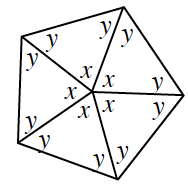### Home > CCG > Chapter Ch8 > Lesson 8.1.1 > Problem8-9

8-9.

Examine the geometric relationships in the diagram at right. Show all of the steps in your solutions for $x$ and $y$.

$x + x + x + x + x = 360º$

$2y + x = 180º$